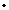Discussion Meeting
ORGANIZERS
Indranil Biswas (TIFR, Mumbai, India) and Mahan Mj (TIFR, Mumbai, India)
DATE & TIME
20 February 2023 to 25 February 2023
VENUE
Ramanujan Lecture Hall and Online

This is a followup discussion meeting on complex and algebraic geometry involving the below speakers.

1. Claire Voisin
Hyper-Kahler manifolds: Yau’s theorem on the existence of Ricci flat Kahler metrics on compact Khler manifolds with trivial canonical bundle has for consequence the Beauville-Bogomolov theorem, which states that, up to an tale cover, they are products of complex tori, Calabi-Yau manifolds in the strict sense, and hyperKhler manifolds, that are also called irreducible symplectic holomorphic manifolds, because their main characterization is the existence of a unique (up to a coefficient) everywhere nondegenerate holomorphic 2-form. The deformation theory of hyper-Kahler manifolds is well-understood using the period map, which studies how the cohomology class of the holomorphic 2-form varies with the complex structure. However the classification is missing and we do not know if there are many topological types of hyper-Kahler manifolds in each even dimension. I will present the main features of this geometry and sketch the proof of a simple topological characterization of the hyper-Kahler fourfolds obtained by deforming Hilb2 (K3) (one of the two topological types we know in dimension 4). This is joint work with O. Debarre, D. Huybrechts, E. Macr.

2. Bruno Klingler
Hodge thoery, between algebraicity and transcendence: Hodge theory, as developed by Deligne and Griffiths, is the main tool for analyzing the geometry and arithmetic of complex algebraic varieties. It is an essential fact that at heart, Hodge theory is NOT algebraic. On the other hand, according to both the Hodge conjecture and the Grothendieck period conjecture, this transcendence is severely constrained. Tame geometry, which studies structures where every definable set has a finite geometric complexity, appears as a natural setting for understanding these constraints: period maps associated to variations of Hodge structures on complex quasi-projective varieties are tame. I will explain how functional transcendence results for period maps in this setting (Ax-Schanuel for variations of Hodge structures) enable us to prove surprisingly strong algebraicity results for Hodge loci. Time permitting, I will turn to the arithmetic aspects of these questions. Based on the work of Bakker, Baldi, Brunebarbe, Ullmo, Tsimerman and myself.

3. Alessandro Ghigi and Paola Frediani
Differential geometry of the Torelli map: Let j : Mg→Ag be the Torelli map. Outside the hyperelliptic locus j is an orbifold embedding. The goal of the lectures is to relate infinitesimal properties of this embedding at a moduli point [C] with the geometry of the curve C. We will first describe the computation of the second fundamental form using Hodge-Gaussian maps. We will next reinterpret it as a multiplication map on sections and we will derive dimension bounds for totally geodesic subvarieties generically contained in the Jacobian locus. We will also describe the connection with the projective structures on Riemann surfaces. Finally we will consider the Lie bracket on the tangent space of T[C]→g and we will show that its restriction to T[C]M∗g can be interpreted as the multiplication map by the Bergman kernel.

programictsresin